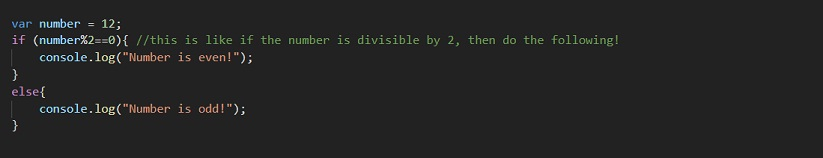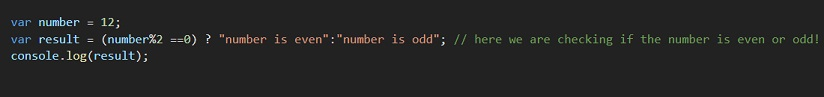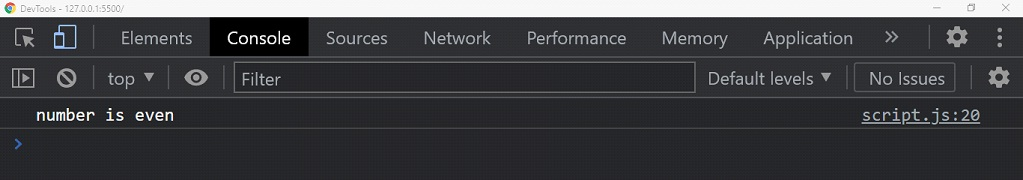# Ternary Operator in JavaScript

Ternary Operator JavaScript

The conditional operator in JavaScript or the ternary operator in JavaScript is kind of a short code for the if-else. You can use this instead of the if-else in some situations.

## Ternary Operator JavaScript

Let’s have a look at the syntax first so that we can get a brief idea about what the ternary operator is –

condition ? exp1 : exp2;

Well, that’s it. This is the syntax of the ternary operator. There is some condition (similar to what we give in the if statement) here, followed by a question mark. Then, we have an expression exp1, which executes if the condition is true. Then we have a colon, and then the expression exp2, which executes if the condition is false.

First, let’s see something with the simple if-else statements, and then we will try to do the same thing with the help of a ternary operator.So, if you try to run the above program, the output that we are going to have is – Number is even!

You can try changing the values and see the changes. Now, this is one way, but we have another way as well. That is, using the ternary operator. Let’s now have an example, so that we can understand the working of the ternary operator.If now we run to the console to see the output, we are going to see something like this –As you can see, this was pretty similar to the if-else thing. We can use this as and when required. It is frequently used in place of the simple if-else. But if there is the nesting of if-else, or there is some complication, we should avoid using the ternary operator, since it may create some sort of confusion. Otherwise, the ternary or conditional operator is very useful.